Function Repository Resource:

# HookLengths

Get the list of lists whose entries are the hook lengths of the entries of a Young tableau

Contributed by: George Beck
 ResourceFunction["HookLengths"][p] gives a list of lists of lengths p for a partition p where an entry is the hook length of the corresponding cell in the Young tableau of p.

## Details

In the Young tableau of shape p, the hook for a cell c is the set of cells containing c, the cells to the right of c and the cells below c.
The hook length of a cell c is the number of cells in the hook of c.

## Examples

### Basic Examples (2)

The hook lengths of the integer partition (3,2,1,1):

 In:=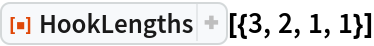Out=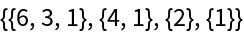Visualize the corresponding Ferrers diagram:

 In:=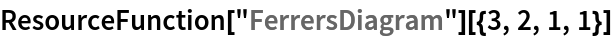Out=### Scope (4)

Here is a partition:

 In:=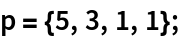The Young diagram with shape p:

 In:=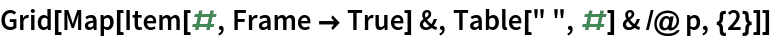Out=A hook of length 5:

 In:=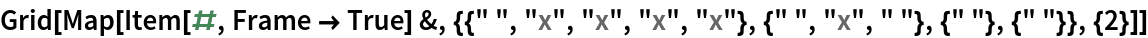Out=The hook lengths of p:

 In:=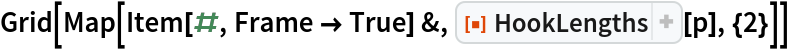Out=### Properties and Relations (3)

Here is a partition:

 In:=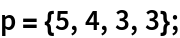The number of standard Young tableaux for the diagram of shape p (the hook length formula):

 In:=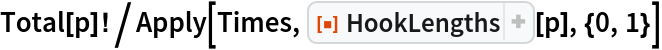Out=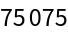This is the same as the result of the resource function NumberOfTableaux:

 In:=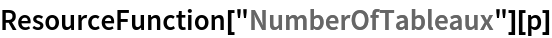Out=George Beck

## Version History

• 2.0.1 – 07 February 2022
• 2.0.0 – 10 July 2019
• 1.0.0 – 18 February 2019